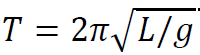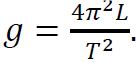BACK

# Science learning in times of competitive entrance examinations

### Summary

Science students in our country are spending increasing effort and time at private coaching institutes training for entrance examinations for higher studies. Instead of looking at this shift merely through a binary framework of formal science learning in schools and colleges versus training for entrance examinations, it is more fruitful to identify learning deficits which are shared across this divide. In this article, Sanjay Kumar identifies three such deficits in physics learning.

It will be wrong to view this shift in students’ time and effort through the binary of formal education in schools and universities versus training for entrance examinations at coaching institutes. It is not as if the science learning will become better if this tilt is somehow arrested and our students  put in more effort at curricula based learning in schools and colleges. In fact, the shift would have been helpful if it complemented science learning in schools and colleges and empowered students to overcome latter’s learning deficits. This however is not happening. Instead, both the domains for science learning suffer from shared deficits. This article explores the specific case of physics learning to find out some of the blind-spots where the science education ecosystem of the country, including both the curricula based learning in schools/colleges and training for entrance examinations, is failing its students. The process of science learning suffers from multi-sectoral failure. Corrective measures will need to be multi-dimensional.

‘Cramming Formulas’: Side Effects of a Practical Key to Success

To recognise the effects of coaching institute based training on students’ science learning, it is essential to appreciate its specific purpose and techniques. Success in most competitive examinations in sciences is now based upon how many questions a student can answer in limited amount of time. Students realise early that speed is the key. To achieve this goal, coaching institutes develop a compendium of all possible problems that can be formulated on a given topic, and then explain to students the quickest ways to solve them. This is a toolkit approach, with a specific tool for every possible problem.

The structural necessity of competitive examinations forces students to go through what one physics student in a conversation called ‘cramming formulas’. A physics formula is a mathematical result of laws of physics applied to conditions of a set of problems. There is simply no time during a competitive examination to derive the relevant formula from basic principles. Coaching institute training provides templates of association between formulas and problems, which presumably serves students well in solving problems quickly.

However, proficiency in figuring out the most suitable formula for a given problem does not imply that a student actually understands the underlying physics. Difficulty arises from the mathematical structure of a formula. A typical formula relates different physical quantities through the relationship of equality. This relationship is  bi-directional; A= B is equivalent to B=A. Since simple mathematical operations allow expressions of all quantities to be moved around in a formula, including across the equality sign, the formal structure of a formula treats all physical quantities equally. Identification of the relevant formula, and proficiency in mathematical operations on it, are the key skills a student develops to generate associative templates that relate a particular formula to a set of problems.

While a correct formula indeed is a powerful tool to solve a problem, and ability to manipulate it an essential skill, these very properties also create hurdles in developing a physics based understanding. Explication of causal relations between physical quantities, and distinction between different levels of generalities are the two key components of explanation and understanding in physics. Causal relations are directional. The meaning of “A causes B” is fundamentally different from “B causes A”. This is the opposite of the meaning of an associative relationship like equality in a formula. Further, the equality of the formal status of all quantities in a formula does not make obvious their different levels of generalities.

The above points can be explained with the help of a simple example. All first year physics undergraduate students study oscillations of a rigid body, during which a formula relating the time period T of oscillations, the acceleration due to gravity (g), and a quantity L called the ‘length of equivalent simple pendulum’ is derived. The formal expression of this formula isStudents also use this formula in a laboratory experiment during which they find acceleration due to Earth’s gravity by turning the formula around asA physics based explanation of the phenomenon can go something like this. A rigid body is free to rotate about an axis of rotation. If disturbed from equilibrium, the force of gravity causes an unbalanced restoring torque which tends to take the body back towards equilibrium. The inertia of rotation causes overshooting from the equilibrium, which leads to a restoring torque in the opposite direction. The time period of oscillations depends upon the acceleration due to gravity and the distance of the axis of rotation from the center of mass of the body. The latter determines L, which enters the formula for T. The explanation is built around causal relationships between different physical quantities: g causes unbalanced torque, inertia of rotation causes overshooting from equilibrium, T depends upon g and L, etc. A comprehensive understanding also requires an appreciation of different levels of generalities. Acceleration due to gravity is the most general. It forms the very environment in which the rigid body can oscillate.  L is a structural feature of the rigid body. It is not an invariant characteristic like its mass. It depends upon the distribution of the mass in the body, and on the axis of rotation. T is the least general. It changes with any alteration in acceleration due to gravity, a change in the distribution of mass in the body, as well as a change of the axis of rotation.

Neither of the two formulas related to the phenomenon give much clue about the causal relations, and the levels of generalities. This can be seen from students’ answers to a question like what will happen if the same rigid body is taken to the Moon and made to oscillate. From their general knowledge they know that the acceleration due to gravity at the Moon is less. However, they cannot to figure out how would L and T behave, just on the basis of their familiarity with the formula.

Sinking in the Sea of Derivations

Physics has evolved a fairly elaborate theoretical structure of fundamental concepts and laws during its evolution in the last four centuries. All physics textbooks, which form the basis of class room teaching and lectures in schools and colleges, describe and explain this structure. A text based learning of physics can be broadly divided in two stages. The first stage is the development of familiarity with the subject’s theoretical structure. The next important step is  a deeper understanding of the subject by working out extensions of the theoretical structure given in the text, and solving conceptual and quantitative problems. Familiarity is a necessary first step, however it does not guarantee success in the next step.

School and college based physics learning in our country rarely goes beyond the first stage of familiarity with its theoretical structure. Classroom teaching is largely limited to exposing students to results already derived in textbooks. Students limit their learning effort to reproducing text based derivations in examinations. There is no doubt that an exposure to subtleties of basic concepts and derivations of essential results does introduce students to modes of scientific reasoning. However, unless students are also encouraged to, and tested for applying this reasoning on their own in solving unfamiliar problems, their cognitive abilities to ‘do’ physics remain limited. In fact the focus on derivations waylays scientific reasoning and encourages the proverbial ‘rote learning’ tendency of the Indian education system.

It might appear that the focus of coaching institutes on training students for solving problems for entrance examinations should help them in the second stage of developing an understanding of the theoretical structure. However, as we saw, the reality is very different. Rather than any deeper understanding, the training for entrance examinations may be leading to shallow ‘misunderstandings’.

Laboratory Work: The Step Child of Science Learning

Laboratory based experiments are part of physics curricula all along the way from secondary school level to post graduate studies. The purpose of laboratory-based learning in science education is two-fold. One, it introduces students to specific experimental physics methods and apparatuses, so that they get trained for doing experimental research later in their scientific careers. The second, in fact the more important purpose, is to help students start  ‘thinking’ about physical objects and processes in terms of concepts and laws of physics. Hence, anybody can see that a pendulum is an oscillating object. A physics student has to develop the ability to think of it as an object under unbalanced forces and torques, figure out how these cause oscillations in conjunction with the property of inertia, and appreciate the fact that the motion can be approximated by a very simple model of simple harmonic motion. This mode of thinking involving abstract concepts and relations while working with observable objects and processes is characteristic of all empirical sciences.

Testing students for laboratory based understanding and skills requires on the spot testing of individual performance. This is not feasible in a nationwide, short duration entrance examination in which hundreds of thousands of student participate at the same time. There is no place for laboratory-based learning in training for entrance examinations. Is the condition any better in our schools and colleges?

The system of laboratory work in our schools is severely stressed. The crisis is most acute in state funded public education. The teaching of laboratory methods, availability of scientific apparatuses, opportunity for students to actually perform experiments are all lacking. Instead of indicating systemic shortfalls, the evaluation system puts it’s stamp of approval on lack of learning. Grade inflation is rampant. Most students get cent-percent marks. Laboratory work in colleges and universities has not yet degenerated to the level of our pubic schools. However, here too laboratory teaching and learning is considered secondary to theoretical learning in lectures. The new Choice Based Credit System mandated by the UGC has structurally formalised this secondary status of laboratory work. A laboratory course is attached to every theory course with half the credits of the theory part. This scheme is driven by the philosophy that the role of laboratory work is only to show students practical applications of what they learn in their theory papers. This completely neglects the independent significance of experimental techniques and methods like instrument design and fabrication, data acquisition and analysis, and error estimation.

Focus on success in entrance examinations demotivates students from laboratory work. A dysfunctional laboratory system in schools and colleges also leads to the same result. The pull and push factors work from both directions to squeeze out an essential component of science learning.

Learning sciences is a complex and unpredictable exercise. The primary responsibility of educational institutions is to provide an enabling environment for this exercise. Goal-specific training for success in entrance examinations alienates students from laboratory work. Formula based problem solving skills do not necessarily help them identify causal relations and different levels of generalities. Formal science education in schools and colleges also leads to the same lacunae. While internal reform in the formal stream of science learning is a must, institutions of higher learning also need to move away from the practice of deciding admissions only on the basis of performance in one examination.

Sanjay Kumar teaches Physics at St Stephen’s College, Delhi. He would like to thank students of St Stephen’s College, Shivaji College, and ARSD College of the University of Delhi for sharing their ideas and experiences. The views expressed are the author’s own.